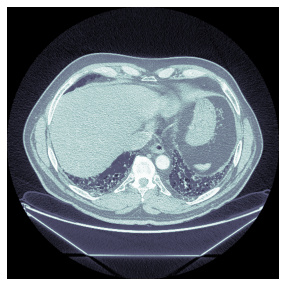# Fastai2: Problems with Medical Images (DICOM)

Hi there,

currently I am working on the OSIC Pulmonary Fibrosis Progression Data. I have loaded the Dicom Files in a datablock and I can display it.

The problem is, that the data is not normalized and I could not figure out how to normalize it. It is extremely bright or dark.

Here is my code so far:

``````root_dir = Path('kaggle/input/osic-pulmonary-fibrosis-progression')

items = get_dicom_files(root_dir/f"train/")

data = DataBlock(blocks=(ImageBlock(cls=PILDicom), CategoryBlock),
get_x= lambda x: x,
splitter=RandomSplitter(seed= 2),
item_tfms = [Resize(size= 512, resamples= (Image.NONE,0))],
batch_tfms = [*aug_transforms(), Normalize.from_stats(*imagenet_stats)])

``````

Now, if I display it by running the following code:

``````dls.show_batch(max_n= 4)
``````

I get the following images:

I would like to get something like this, where the normalization is applied:Which I get, when I run the following code:

``````items.dcmread().show()
``````

Any idea how I can do that?

Thank you!

P.S.:

I had to use the following parameter in the Resize method:

`````` resamples= (Image.NONE,0)
``````

Otherwise I would get a ValueError: “Image has wrong mode”.

1 Like

The `dcmread().show()` function has the following signature:

``````dimg.show(
scale=True,
cmap=<matplotlib.colors.LinearSegmentedColormap object at 0x7f5d0c182f10>,
min_px=-1100,
max_px=None,
ax=None,
figsize=None,
title=None,
ctx=None,
norm=None,
aspect=None,
interpolation=None,
alpha=None,
vmin=None,
vmax=None,
origin=None,
extent=None,
filternorm=1,
resample=None,
url=None,
*,
data=None,
)
``````

From this I was able to deduce that the cmap is `plt.cm.bone`, so you can start by using `dls.show_batch(cmap=plt.cm.bone)`.

This gets you halfway there, but you probably need to also account for: `min_px=-1100`, `filternorm=1` and `filterrad=4.0`. I’m guessing this could be done using `item_tfms`, but requires some additional research.

Please let me know if you make any progress, I think we are in a similar position.

ValueError: “Image has wrong mode” might be due to this issue https://github.com/python-pillow/Pillow/issues/4402. The Resize method in fastai uses bicubic interpolation per default which apparently does not work for uint16 images.

I ran into similar problems with medical images. When working with DICOM images one has to differentiate between the raw pixel values and how the image will be displayed, the later is specified in the image header. However, the image header is not used by `dcmread`. Also, as Martin correctly said, DICOM are often uint16, which is not supported by PIL.
To effectively work with DICOM I slightly adapted the `PILDicom.create` and `dcmread` function.

``````def dcmread2(fn):
intercept=dcm[0x0028, 0x1052].value
slope=dcm[0x0028, 0x1053].value
arr=dcm.pixel_array
return intercept + arr*slope
``````

The `dcmread2` function rescales the DICOM image before returning the pixel array (see here for more information). Rescale intercept and slope are both given in the header. Rescaling might be important for both CT and X-ray, as the raw pixel data can be very different from rescaled data. For MRI it is usually not of much importance. However, be careful with CT as negative values will appear after after rescaling.

Next is to adapt `PILDicom` to use `dcmread2` and also convert pixel data to uint32, which can be handled by PIL.

``````class PILDicom2(PILBase):
"same as PILDicom but changed pixel type to np.int32 as np.int16 cannot be handled by PIL"
_open_args,_tensor_cls,_show_args = {},TensorDicom,TensorDicom._show_args
@classmethod
def create(cls, fn:(Path,str,bytes), mode=None)->None:
"Open a `DICOM file` from path `fn` or bytes `fn` and load it as a `PIL Image`"
if isinstance(fn,(Path,str)): im = Image.fromarray(dcmread2(fn).astype(np.int32)) # images are np.int16, but this cannont be handled by PIL. Will throw wrong mode error.
im = im._new(im.im)
return cls(im.convert(mode) if mode else im)
``````

Lastly, when construction the datablocks, the `batch_tfms` need to be adapted:

``````data = DataBlock(
...
batch_tfms=[IntToFloatTensor(div=2**16-1), *aug_transforms()])
``````

Per default `IntToFloatTensor` will divide by 255, but as the DICOM images are originally 16 Bit, pixel values can be up to 2^16. `IntToFloatTensor` will clip all values below 0 or above 1, this is the reason you image display look so strange.

Hope this helps

5 Likes